Ex 6.1

Chapter 6 Class 10 Triangles
Serial order wise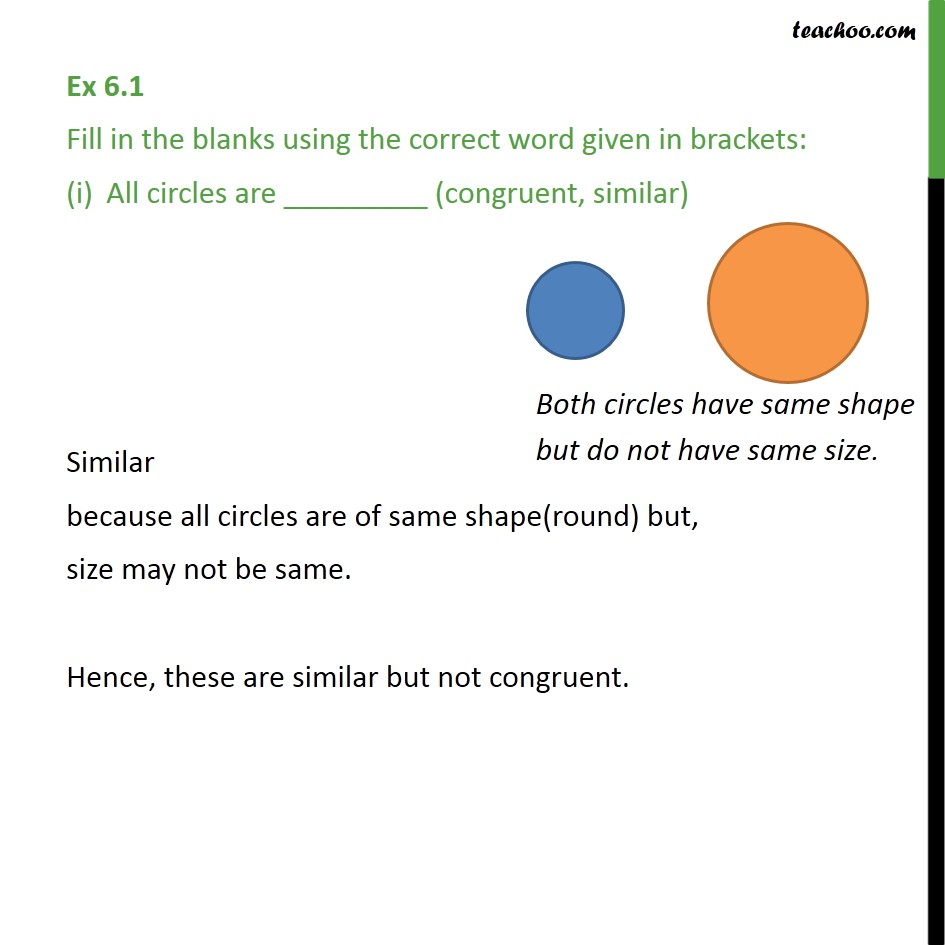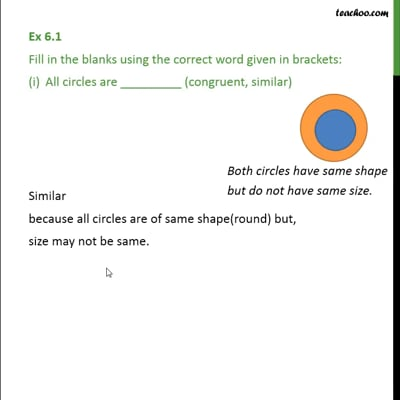This video is only available for Teachoo black users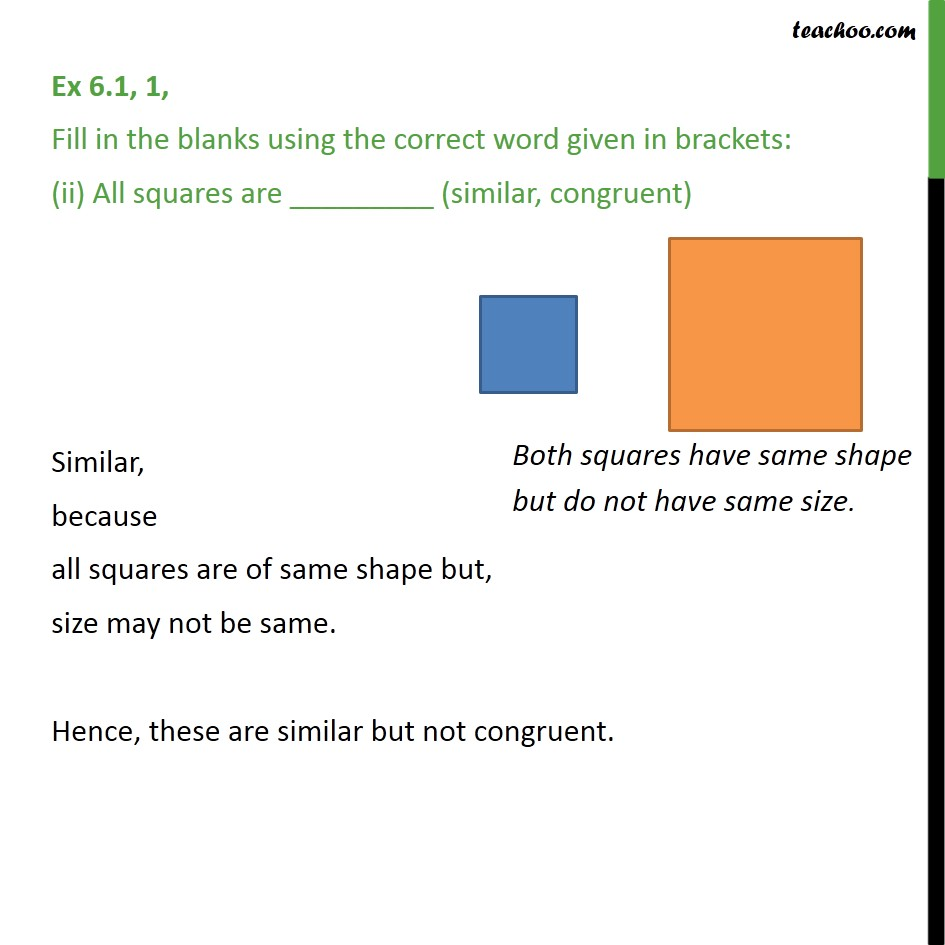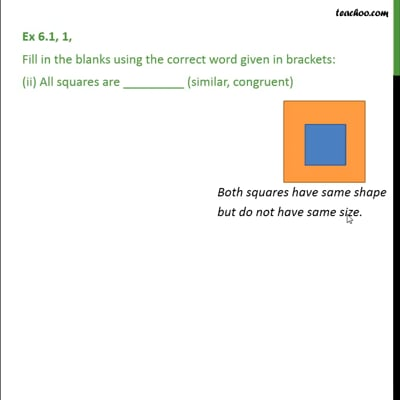This video is only available for Teachoo black users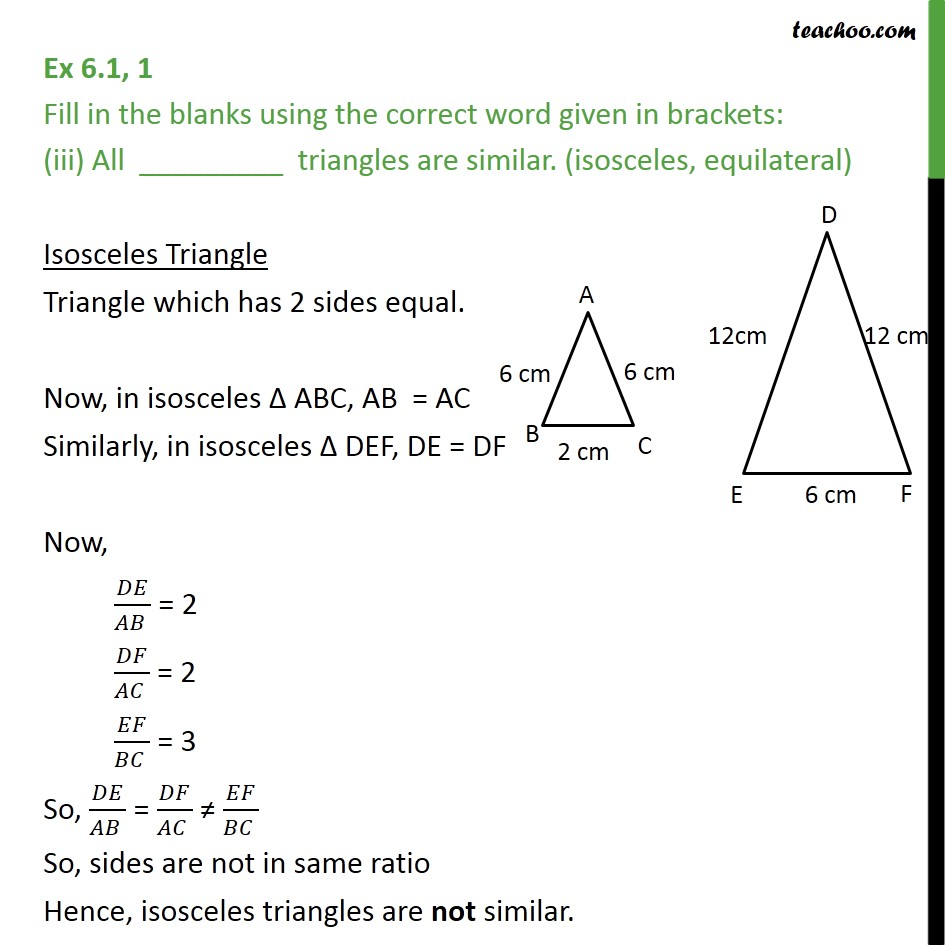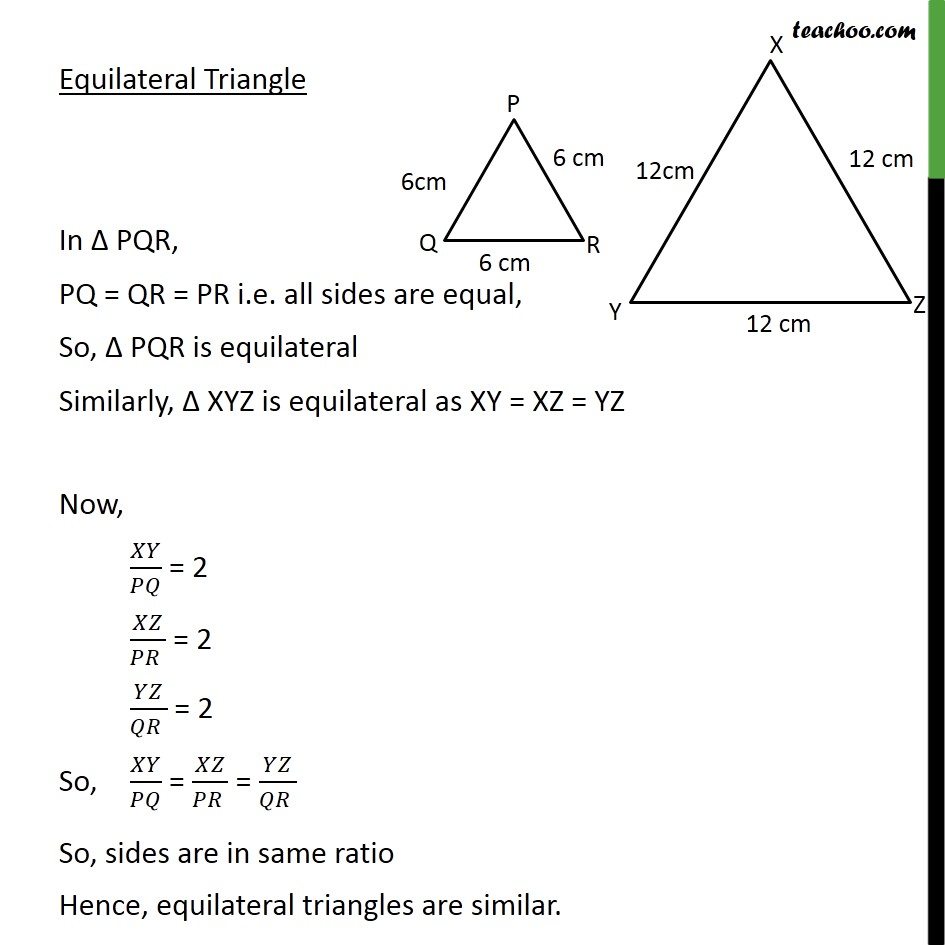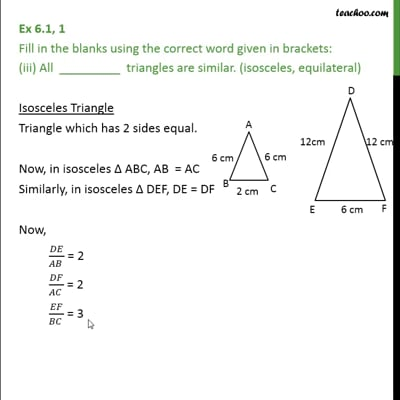This video is only available for Teachoo black users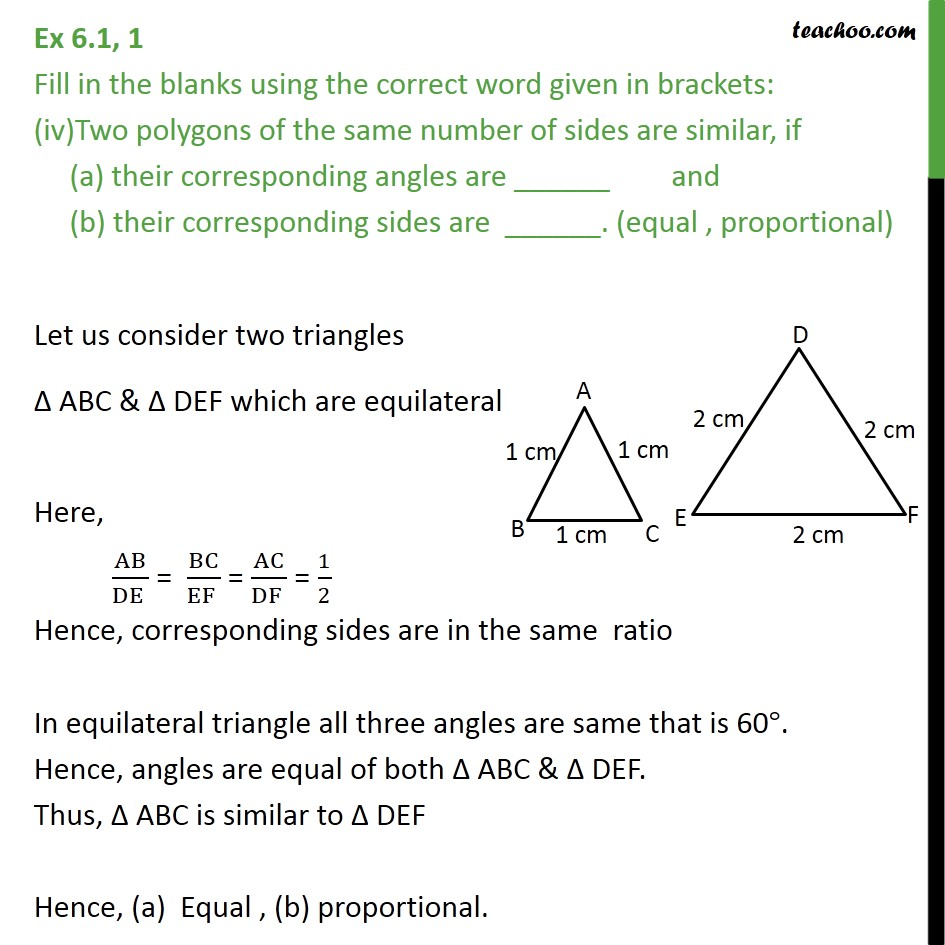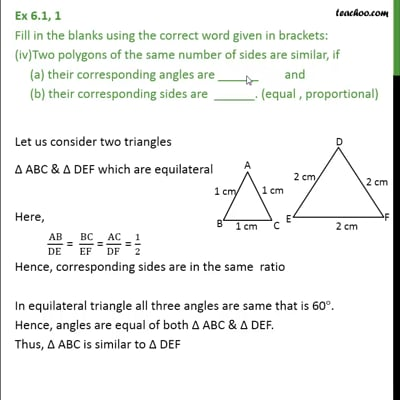This video is only available for Teachoo black users

Maths Crash Course - Live lectures + all videos + Real time Doubt solving!

### Transcript

Ex 6.1 Fill in the blanks using the correct word given in brackets: All circles are _________ (congruent, similar) Similar because all circles are of same shape(round) but, size may not be same. Hence, these are similar but not congruent. Ex 6.1, 1, Fill in the blanks using the correct word given in brackets: (ii) All squares are _________ (similar, congruent) Similar, because all squares are of same shape but, size may not be same. Hence, these are similar but not congruent. Ex 6.1, 1 Fill in the blanks using the correct word given in brackets: (iii) All _________ triangles are similar. (isosceles, equilateral) Isosceles Triangle Triangle which has 2 sides equal. Now, in isosceles ABC, AB = AC Similarly, in isosceles DEF, DE = DF Now, /( ) = 2 /( ) = 2 /( ) = 3 So, /( ) = /( ) /( ) So, sides are not in same ratio Hence, isosceles triangles are not similar. Equilateral Triangle In PQR, PQ = QR = PR i.e. all sides are equal, So, PQR is equilateral Similarly, XYZ is equilateral as XY = XZ = YZ Now, / = 2 /( ) = 2 /( ) = 2 So, / = /( ) = /( ) So, sides are in same ratio Hence, equilateral triangles are similar. Ex 6.1, 1 Fill in the blanks using the correct word given in brackets: Two polygons of the same number of sides are similar, if (a) their corresponding angles are ______ and (b) their corresponding sides are ______. (equal , proportional) Let us consider two triangles ABC & DEF which are equilateral Here, AB/(DE ) = BC/(EF ) = AC/(DF ) = 1/2 Hence, corresponding sides are in the same ratio In equilateral triangle all three angles are same that is 60 . Hence, angles are equal of both ABC & DEF. Thus, ABC is similar to DEF Hence, (a) Equal , (b) proportional.